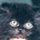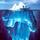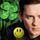## General Question# Could someone explain to me the frequency domain in physics?

Asked by sunssi (120) May 31st, 2011

I’m trying to learn fourier transform but I dont get how the time domain is displayed as the frequency domain.

Don’t explain the fourier transform

Observing members: 0Composing members: 0## 2 AnswersYou regard a function of time as if it were a periodic wave, representing the superposition of multiple sine waves. Each sine wave has a different frequency, amplitude, and phase shift, so the whole mixture is a kind of “recipe” for re-creating the original function.

For actual periodic waves it’s called Fourier analysis, where there are discrete frequencies called overtones or harmonics. Fourier transforms, on the other hand, can handle an arbitrary function (especially one of finite time duration) using continuous rather than discrete frequencies.

Thus a function of time is Fourier-transformed into a function of frequency, representing the unique mix of sine waves that, all superimposed together, forms the original time-domain function. It’s pretty remarkable.

Here “domain” is in the sense of the x-coordinate of a mathematical function y = f(x). In time domain (what you see on an oscilloscope, for instance) the horizontal axis is time. In frequency domain the horizontal axis is frequency.

There is a symmetric duality between time domain and frequency domain—they are flip sides of the same function. Engineering problems sometimes are more easily solved by working the frequency domain instead of the time domain. Imagine an audio engineer setting EQ on a recording—that frequency-domain stuff.

The only catch is that Fourier transforms are usually complex numbers with both real and imaginary components, requiring an extra dimension to visualize. So often the frequency-domain representation shows only the magnitude or power of each frequency, discarding some of the phase information.

gasman (11296)“Great Answer” (6) Flag as…I’ll give you an example. Imagine the musical note “A” . It is 440 Hz. Now think, how would you plot it on a graph? You could make a plot of pressure vs. time. That would look like a sine wave that wiggles at 440 Hz. The louder it is, the taller. Let’s add another wave to it. Make it 1000Hz. That would be superimposed on top of the first wave making it a little difficult to see. That is the time domain.
Let’s make it easier to see. Make the X axis frequency and the Y axis power.
Te plot is real easy. It would have one spike at 440 Hz and another at 1000 Hz. That is the frequency domain.
Look at the graphic equalizer on your stereo. That is also the frequency domain. You can see the high and low frequencies and see how much bass is booming. If you were looking in the time domain it would just be a mush of lines.

LuckyGuy (36619)“Great Answer” (3) Flag as…## Answer this questionor

#### Join

to answer.

This question is in the General Section. Responses must be helpful and on-topic.

Your answer will be saved while you login or join.
Have a question? Ask Fluther!
What do you know more about?
or
Knowledge Networking @ Fluther